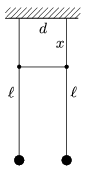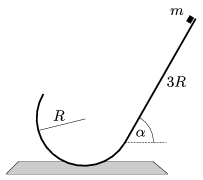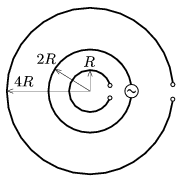Mathematical and Physical Journal
for High Schools
Issued by the MATFUND Foundation
 Already signed up? New to KöMaL?

# KöMaL Problems in Physics, January 2011

Show/hide problems of signs:## Problems with sign 'M'

Deadline expired on February 10, 2011.

M. 311. Make a connection between two simple pendulums of the same lengths, with a wooden skewer, which is attached to the threads. Displace one of the pendulums in the plane determined by the two pendulums and measure how long it takes for this pendulum to stop and for the other to swing with the greatest amplitude. How does this time depends on the distance denoted by x shown in the figure?(6 pont)

statistics## Problems with sign 'P'

Deadline expired on February 10, 2011.

P. 4305. Why do transparent objects, like glasses, have shadows?

(3 pont)

solution (in Hungarian), statistics

P. 4306. It is a well known fact, that it is easier to reach the same speed with a bicycle in tailwind and harder in headwind than in calm weather. What happens when the direction of the wind is perpendicular to the direction of the motion?

(4 pont)

solution (in Hungarian), statistics

P. 4307. Two pointlike elastic balls, which have the same mass, are projected vertically upward with the same initial speed; the second ball is started 4 s later than the first one. a) What will the velocities of the two balls be right before and after the first collision? (Air drag can be neglected.) b) Determine and graph the distance between the position of the first collision and the ground as a function of the initial speed.

(4 pont)

solution (in Hungarian), statistics

P. 4308. The bottom end of a smooth inclined plane of angle of elevation=60o, and of length 3R is attached to a cylinder, which has a semicircular cross section of radius R=0.5 m. In the path there is no break. From the top of the inclined plane a pointlike object of mass m=0.2 kg slides down with zero initial speed. a) What will the kinetic energy of the object be at the highest point of the curved part of the path? b) Where will the object hit the inclined plane? (Friction and air drag are negligible.)(5 pont)

solution (in Hungarian), statistics

P. 4309. There is some water in a container which is on a table. The bottom of the container is connected with a tube to the interior part of a black box which is next to the table and which is as big as a cupboard. If a little more water is poured into the container, then the original level of the water decreases, and if some water is taken out of the container the level of water in it increases. What can be in the black box?

(5 pont)

solution (in Hungarian), statistics

P. 4310. An air gun is modelled such that initially there is some compressed air in a container of volume V1, at a pressure of p1. In the barrel there is a small projectile of mass m right in front of the container's opening. a) How long should the barrel be in order to accelerate the projectile to the greatest speed? b) What can the maximum speed of the projectile be? (Neglect friction and the leaking of the high-pressure air.) Data: m=0.51 g, the inner diameter of the barrel is 4.5 mm, p1=2 MPa, V1=1 cm3.

(5 pont)

solution (in Hungarian), statistics

P. 4311. Consider a classroom at a temperature of 25 oC in which the air pressure is the normal atmospheric pressure. Estimate the distance (in nm) between a) two nearby Oxygen molecules; b) two nearby Nitrogen molecules.

(4 pont)

solution (in Hungarian), statistics

P. 4312. Three open conductor rings, made of thin wire, are placed concentrically on the horizontal tabletop as shown in the figure. The current which flows in the middle ring is varied in time. Find the induced emf in the greatest ring when the induced emf between the ends of the smallest ring is U0.(6 pont)

solution (in Hungarian), statistics

P. 4313. Subscribers can reach the text of the problem after signing in. The text will be public from January 13, 2011.]

(4 pont)

solution (in Hungarian), statistics

P. 4314. In 1923 György Hevesy determined the age of a piece of rock which contained Uranium. For this he measured the ratio of the atoms of 238U to the atoms of 206Pb in the rock, which was 2:3. How old did he find the rock? The half-life of Uranium is 4.51.109 years.

(4 pont)

solution (in Hungarian), statistics